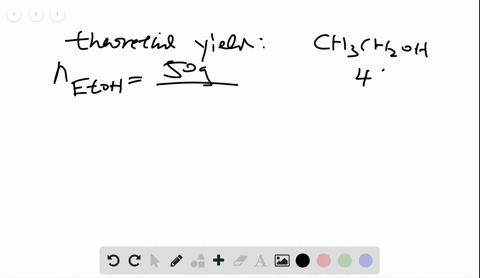🎉 The Study-to-Win Winning Ticket number has been announced! Go to your Tickets dashboard to see if you won! 🎉View Winning Ticket## Discussion

You must be signed in to discuss.

## Video Transcript

given the following reaction, we can figure out how to create a solution that has 0.511% lead chrome eight per kilogram of paint solution, looking at our initial compound as well as the replacement of the potassium by the lead in the final substance. So if we have 0.511% lead chrome eight in a kilogram or 1000 grams, that means that we're looking for 5.11 grams of lead chrome eight. So we'll do a conversion from grams of lead chromite, which will change two moles. And we do that using the molar Mass. And then we could change from moles of lead, chrome eight two moles, a potassium, chrome eight. And we can do that by looking at the formulas of the ionic compounds in the ratio of ions. Once we know that we can then use our equation to go to moules of F E. C. R. Four using the mole ratio from the balanced equation and then finally find out how many grams that's equivalent to using the molar mass. So 0.5 11% is 5.11 grams of PBC roo for which we change. Two moles using the Moeller math 3300 and 23.19 grams. We can see that for every lead there's one potassium chrome eight because of the chromite ions and then using that information, we see that for every eight moules okay to see our for therefore moles the iron C R 04 And then finally we multiply by the molar mass, which is 223.83 grams per mole. So multiplying 5.11 times four times 200 Cray 3.83 dividing by 323.19 times eight. We see that there should be 1.77 grams of Effie c r 04 to make the paint.